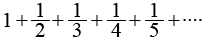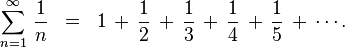# Python: Recursion - Exercises, Practice, Solution

## Recursion [ 11 exercises with solution]

[An editor is available at the bottom of the page to write and execute the scripts.]

1. Write a Python program to calculate the sum of a list of numbers. Go to the editor
Click me to see the sample solution

2. Write a Python program to converting an Integer to a string in any base. Go to the editor
Click me to see the sample solution

3. Write a Python program of recursion list sum. Go to the editor
Test Data: [1, 2, [3,4], [5,6]]
Expected Result: 21
Click me to see the sample solution

4. Write a Python program to get the factorial of a non-negative integer. Go to the editor
Click me to see the sample solution

5. Write a Python program to solve the Fibonacci sequence using recursion. Go to the editor
Click me to see the sample solution

6. Write a Python program to get the sum of a non-negative integer. Go to the editor
Test Data:
sumDigits(345) -> 12
sumDigits(45) -> 9
Click me to see the sample solution

7. Write a Python program to calculate the sum of the positive integers of n+(n-2)+(n-4)... (until n-x =< 0). Go to the editor
Test Data:
sum_series(6) -> 12
sum_series(10) -> 30
Click me to see the sample solution

8. Write a Python program to calculate the harmonic sum of n-1. Go to the editor
Note: The harmonic sum is the sum of reciprocals of the positive integers.
Example :Click me to see the sample solution

9. Write a Python program to calculate the geometric sum of n-1. Go to the editor
Note: In mathematics, a geometric series is a series with a constant ratio between successive terms.
Example :Click me to see the sample solution

10. Write a Python program to calculate the value of 'a' to the power 'b'. Go to the editor
Test Data :
(power(3,4) -> 81
Click me to see the sample solution

11. Write a Python program to find  the greatest common divisor (gcd) of two integers. Go to the editor
Click me to see the sample solution

Python Code Editor:

More to Come !

Do not submit any solution of the above exercises at here, if you want to contribute go to the appropriate exercise page.

Test your Python skills with w3resource's quiz

﻿

## Python: Tips of the Day

How do I sort a dictionary by value?

Python 3.6+

```x = {1: 2, 3: 4, 4: 3, 2: 1, 0: 0}
{k: v for k, v in sorted(x.items(), key=lambda item: item)}
{0: 0, 2: 1, 1: 2, 4: 3, 3: 4}
```

Older Python

It is not possible to sort a dictionary, only to get a representation of a dictionary that is sorted. Dictionaries are inherently orderless, but other types, such as lists and tuples, are not. So you need an ordered data type to represent sorted values, which will be a list-probably a list of tuples.

```import operator
x = {1: 2, 3: 4, 4: 3, 2: 1, 0: 0}
sorted_x = sorted(x.items(), key=operator.itemgetter(1))
```

sorted_x will be a list of tuples sorted by the second element in each tuple. dict(sorted_x) == x.

And for those wishing to sort on keys instead of values:

```import operator
x = {1: 2, 3: 4, 4: 3, 2: 1, 0: 0}
sorted_x = sorted(x.items(), key=operator.itemgetter(0))
```

In Python3 since unpacking is not allowed  we can use

```x = {1: 2, 3: 4, 4: 3, 2: 1, 0: 0}
sorted_x = sorted(x.items(), key=lambda kv: kv)
```

If you want the output as a dict, you can use collections.OrderedDict:

```import collections

sorted_dict = collections.OrderedDict(sorted_x)
```

Ref: https://bit.ly/3dJyjpV